February 2011
Mon Tue Wed Thu Fri Sat Sun
« Jan   Mar »
123456
78910111213
14151617181920
21222324252627
28

## Non-Hermitian Perturbations to the Fritzsch Textures of Lepton and Quark Mass Matrices

Harald Fritzsch, Zhi-zhong Xing, Ye-Ling Zhou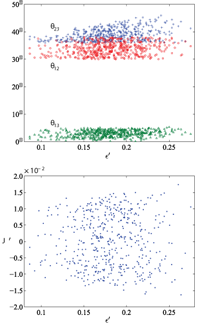We show that non-Hermitian and nearest-neighbor-interacting perturbations to the Fritzsch textures of lepton and quark mass matrices can make both of them fit current experimental data very well. In particular, we obtain \theta_{23} \simeq 45^\circ for the atmospheric neutrino mixing angle and predict \theta_{13} \simeq 3^\circ to 6^\circ for the smallest neutrino mixing angle when the perturbations in the lepton sector are at the 20% level. The same level of perturbations is required in the quark sector, where the Jarlskog invariant of CP violation is about 3.7 \times 10^{-5}. In comparison, the strength of leptonic CP violation is possible to reach about 1.5 \times 10^{-2} in neutrino oscillations.

http://arxiv.org/abs/1101.4272
High Energy Physics – Phenomenology (hep-ph)

## Implementation and testing of Lanczos-based algorithms for Random-Phase Approximation eigenproblems

Myrta Grüning, Andrea Marini, Xavier Gonze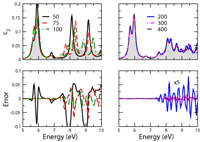The treatment of the Random-Phase Approximation Hamiltonians, encountered in different frameworks, like Time-Dependent Density Functional Theory or Bethe-Salpeter equation, is complicated by their non-Hermicity. Compared to their Hermitian Hamiltonian counterparts, computational methods for the treatment of non-Hermitian Hamiltonians are often less efficient and less stable, sometimes leading to the breakdown of the method. Recently [Gruning et al. Nano Lett. 8, 2820 (2009)], we have identified that such Hamiltonians are usually pseudo-Hermitian. Exploiting this property, we have implemented an algorithm of the Lanczos type for random-Phase Approximation Hamiltonians that benefits from the same stability and computational load as its Hermitian counterpart, and applied it to the study of the optical response of carbon nanotubes. We present here the related theoretical grounds and technical details, and study the performance of the algorithm for the calculation of the optical absorption of a molecule within the Bethe-Salpeter equation framework.

http://arxiv.org/abs/1102.3909
Materials Science (cond-mat.mtrl-sci); Mathematical Physics (math-ph)

## The effective Hamiltonian for thin layers with non-Hermitian Robin-type boundary conditions

Denis Borisov, David Krejcirik

The Laplacian in an unbounded tubular neighbourhood of a hyperplane with non-Hermitian complex-symmetric Robin-type boundary conditions is investigated in the limit when the width of the neighbourhood diminishes. We show that the Laplacian converges in a norm resolvent sense to a self-adjoint Schroedinger operator in the hyperplane whose potential is expressed solely in terms of the boundary coupling function. As a consequence, we are able to explain some peculiar spectral properties of the non-Hermitian Laplacian by known results for Schroedinger operators.

http://arxiv.org/abs/1102.5051
Spectral Theory (math.SP); Mathematical Physics (math-ph)

## Optical Spectral Singularities as Threshold Resonances

Spectral singularities are among generic mathematical features of complex scattering potentials. Physically they correspond to scattering states that behave like zero-width resonances. For a simple optical system, we show that a spectral singularity appears whenever the gain coefficient coincides with its threshold value and other parameters of the system are selected properly. We explore a concrete realization of spectral singularities for a typical semiconductor gain medium and propose a method of constructing a tunable laser that operates at threshold gain.

http://arxiv.org/abs/1102.4695
Optics (physics.optics); High Energy Physics – Theory (hep-th); Mathematical Physics (math-ph); Quantum Physics (quant-ph)

## Periodic orbits for classical particles having complex energy

Alexander G. Anderson, Carl M. Bender, Uriel I. Morone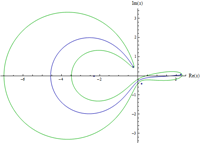This paper revisits earlier work on complex classical mechanics in which it was argued that when the energy of a classical particle in an analytic potential is real, the particle trajectories are closed and periodic, but that when the energy is complex, the classical trajectories are open. Here it is shown that there is a discrete set of eigencurves in the complex-energy plane for which the particle trajectories are closed and periodic.

http://arxiv.org/abs/1102.4822
Mathematical Physics (math-ph); High Energy Physics – Theory (hep-th)

## Making the Case for Conformal Gravity

Philip D. MannheimWe review some recent developments in the conformal gravity theory that has been advanced as a candidate alternative to standard Einstein gravity. As a quantum theory the conformal theory is both renormalizable and unitary, with unitarity being obtained because the theory is a $PT$ symmetric rather than a Hermitian theory. We show that in the theory there can be no a priori classical curvature, with all curvature having to result from quantization. In the conformal theory gravity requires no independent quantization of its own, with it being quantized solely by virtue of its being coupled to a quantized matter source. Moreover, because it is this very coupling that fixes the strength of the gravitational field commutators, the gravity sector zero-point energy density and pressure fluctuations are then able to identically cancel the zero-point fluctuations associated with the matter sector. In addition, we show that when the conformal symmetry is spontaneously broken, the zero-point structure automatically readjusts so as to identically cancel the cosmological constant term that dynamical mass generation induces. We show that the macroscopic classical theory that results from the quantum conformal theory incorporates global physics effects that provide for a detailed accounting of a comprehensive set of 110 galactic rotation curves with no adjustable parameters other than the galactic mass to light ratios, and with the need for no dark matter whatsoever. With these global effects eliminating the need for dark matter, we see that invoking dark matter in galaxies could potentially be nothing more than an attempt to describe global physics effects in purely local galactic terms. Finally, we review some recent work by ‘t Hooft in which a connection between conformal gravity and Einstein gravity has been found.

http://arxiv.org/abs/1101.2186
High Energy Physics – Theory (hep-th); Cosmology and Extragalactic Astrophysics (astro-ph.CO); General Relativity and Quantum Cosmology (gr-qc)

## Armchair graphene nanoribbons: PT-symmetry breaking and exceptional points without dissipation

Maurizio Fagotti, Claudio Bonati, Demetrio Logoteta, Paolo Marconcini, Massimo Macucci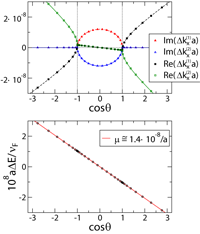We consider a single layer graphene nanoribbon with armchair edges in a longitudinally constant external potential and point out that its transport properties can be described by means of an effective non-Hermitian Hamiltonian. We show that this system has some features typical of dissipative systems, namely the presence of exceptional points and of PT-symmetry breaking, although it is not dissipative.

http://arxiv.org/abs/1102.2129v1
Mesoscale and Nanoscale Physics (cond-mat.mes-hall); Quantum Physics (quant-ph)

## PT-Symmetric Oligomers: Analytical Solutions, Linear Stability and Nonlinear Dynamics

K. Li, P.G. Kevrekidis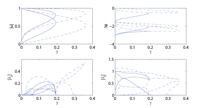In the present work we focus on the case of (few-site) configurations respecting the PT-symmetry. We examine the case of such “oligomers” with not only 2-sites, as in earlier works, but also the cases of 3- and 4-sites. While in the former case of recent experimental interest, the picture of existing stationary solutions and their stability is fairly straightforward, the latter cases reveal a considerable additional complexity of solutions, including ones that exist past the linear PT-breaking point in the case of the trimer, and more complex, even asymmetric solutions in the case of the quadrimer with nontrivial spectral and dynamical properties. Both the linear stability and the nonlinear dynamical properties of the obtained solutions are discussed.

http://arxiv.org/abs/1102.0809
Pattern Formation and Solitons (nlin.PS); Quantum Gases (cond-mat.quant-gas)

## PT-symmetry breaking and laser-absorber modes in optical scattering systems

Y. D. Chong, Li Ge, A. Douglas Stone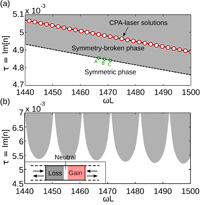Using a scattering matrix formalism, we derive the general scattering properties of optical structures that are symmetric under a combination of parity and time-reversal (PT). We demonstrate the existence of a transition beween PT-symmetric scattering eigenstates, which are norm-preserving, and symmetry-broken pairs of eigenstates exhibiting net amplification and loss. The system proposed by Longhi, which can act simultaneously as a laser and coherent perfect absorber, occurs at discrete points in the broken symmetry phase, when a pole and zero of the S-matrix coincide.

http://arxiv.org/abs/1008.5156
Optics (physics.optics); Quantum Physics (quant-ph)

## Non-Hermitian Euclidean random matrix theory

A. Goetschy, S.E. SkipetrovWe develop a theory for the eigenvalue density of arbitrary non-Hermitian Euclidean matrices. Closed equations for the resolvent and the eigenvector correlator are derived. The theory is applied to the random Green’s matrix relevant to wave propagation in an ensemble of point-like scattering centers. This opens a new perspective in the study of wave diffusion, Anderson localization, and random lasing.

http://arxiv.org/abs/1102.1850
Disordered Systems and Neural Networks (cond-mat.dis-nn); Statistical Mechanics (cond-mat.stat-mech)